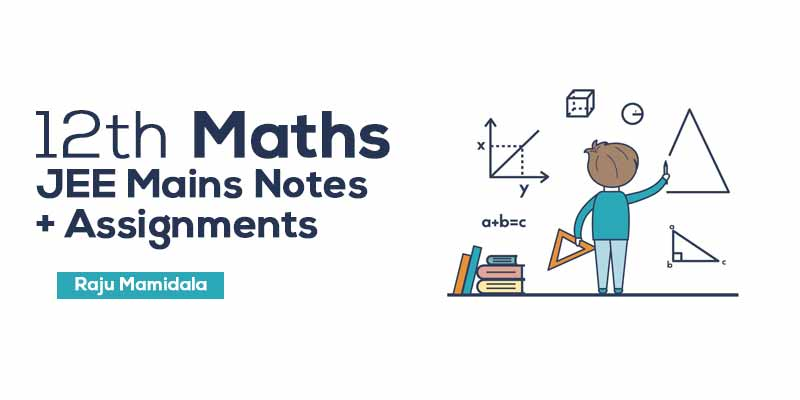## by Raju Mamidala

4.6 (71 Users)
JEE Mains , Maths
Board: CBSE & State Boards , Language: English

Mode- Online
Study Notes- 38
Validity- Till 31 Mar 2020
Language- English
No. of Views- Unlimited

## WHAT WILL I LEARN?

We aim to prepare this class students with a complete study material so that the students can score better in the Mains exam.

Simple and easy to understand.

Understand the level of IIT & JEE concepts by these quick revision notes.

Students can test themselves with assignments which are provided here.

## Curriculum

Binomial theorem

Circles

Areas

• Areas - Class Notes
• Areas - Work Assignment

Probability

• Probability - Class Notes & Work Assignment

Determinants

• Determinants - Class Notes & Work Assignment

Ellipse

• Ellipse - Class Notes
• Ellipse - Work Assignment

Exponential

• Exponential - Class Notes & Work Assignment

hyperbola

• hyperbola - Class Notes
• hyperbola - Work Assignment

linear equations

• linear equations - Class Notes & Work Assignment

mathematical reasoning

• mathematical reasoning - Class Notes & Work Assignment

numerical integration

• numerical integration - Class Notes
• numerical integration - Work Assignment

parabola

• parabola - Class Notes
• parabola - Work Assignment

partial fractions

• partial fractions - Class Notes & Work Assignment

Permutations and combinations

• Permutations and combinations - Class Notes
• Permutations and combinations - Work Assignment

polar coordinates

• polar coordinates - Class Notes
• polar coordinates - Work Assignment

probability

• probability - Class Notes
• probability - Work Assignment

• Quadratic equations - Class Notes
• Quadratic equations - Work Assignment

random variables

• random variables - Class Notes
• random variables - Work Assignment

sets and relations

• sets and relations - Class Notes & Work Assignment

Statistics

• Statistics - Class Notes & Work Assignment

system of circle

• system of circle - Class Notes & Work Assignment

theory of equation

• theory of equation - Class Notes
• theory of equation - Work Assignment

mean value theorem

• mean value theorem - Class Notes & Work Assignment

straight lines

• straight lines - Class Notes & Work Assignment### Raju Mamidala

Mathematics | Class 6th to Competitive exams | 15+ Years Experience | Working in Geethanjali International School in Hyderabad from past 5 years. He Created an Educational Channel for improvement in various aspects for students in IIT Foundation.

## Student's Feedback## 600 399

Includes 5 % GST

Mode- Online

Study Notes- 38

Validity- Till 31 Mar 2020

Language- English

No. of Views- Unlimited